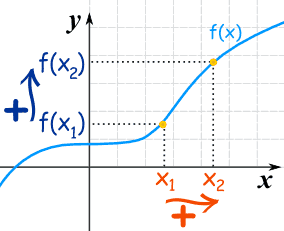# Let f : [0, ∞) → [0, ∞) and g : [0, ∞) → [0, ∞) benon-increasing and non-decreasing functions andh(x) = g[f(x)]. If f and g are differentiable for allpoints in their respective domains and h(0) = 0,then the value of h(3) is……11 years ago

Dear student,

## Increasing Functions

A function is "increasing" if the y-value increases as the x-value increases, like this:It is easy to see that y=f(x) tends to go up as it goes along.

### Flat ?

What about that flat bit near the start? Is that OK?

• Yes, it is OK if you say the function is Increasing
• But it is not OK if you say the function is Strictly Increasing (no flatness allowed)

### Using Algebra

What if you can't plot the graph to see if it is increasing? In that case is is good to have a definition using algebra.

For a function y=f(x):

 when x1 < x2 then f(x1) ≤ f(x2) Increasing when x1 < x2 then f(x1) < f(x2) Strictly Increasing